# 模型解释-LIME的原理和实现2019年9月23日07:01:20

3364字阅读11分12秒

## LIME的原理

LIME的想法很简单, 我们希望使用简单的模型来对复杂的模型进行解释. 这里简单的模型可以是线性模型, 因为我们可以通过查看线性模型的系数大小来对模型进行解释. 在这里, LIME只会对每一个样本进行解释(explain individual predictions).

LIME会产生一个新的数据集(这个数据集我们是通过对某一个样本数据进行变换得到), 接着在这个新的数据集上, 我们训练一个简单模型(容易解释的模型), 我们希望简答模型在新数据集上的预测结果和复杂模型在该数据集上的预测结果是相似的. 我们可以将我们的问题表达为下面的表达式: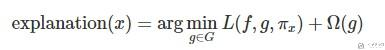• f表示原始的模型, 即需要解释的模型.
• g表示简单模型, G是简单模型的一个集合, 如所有可能的线性模型.
• Pi_x表示我们新数据集中的数据x'与原始数据instance x的距离.
• Ω(g)表示模型g的复杂程度.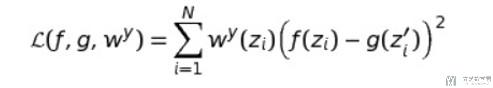• 选择我们想要解释的变量x.
• 对数据集中的数据进行扰动得到新的数据, 同时计算出黑盒模型对这些新的数据的预测值.
• 对这些新的sample求出权重, 这个权重是这些数据点与我们要解释的数据之间的距离.
• 根据上面新的数据集, 预测值和权重, 训练出模型g
• 通过模型g来对模型f在x点附近进行解释.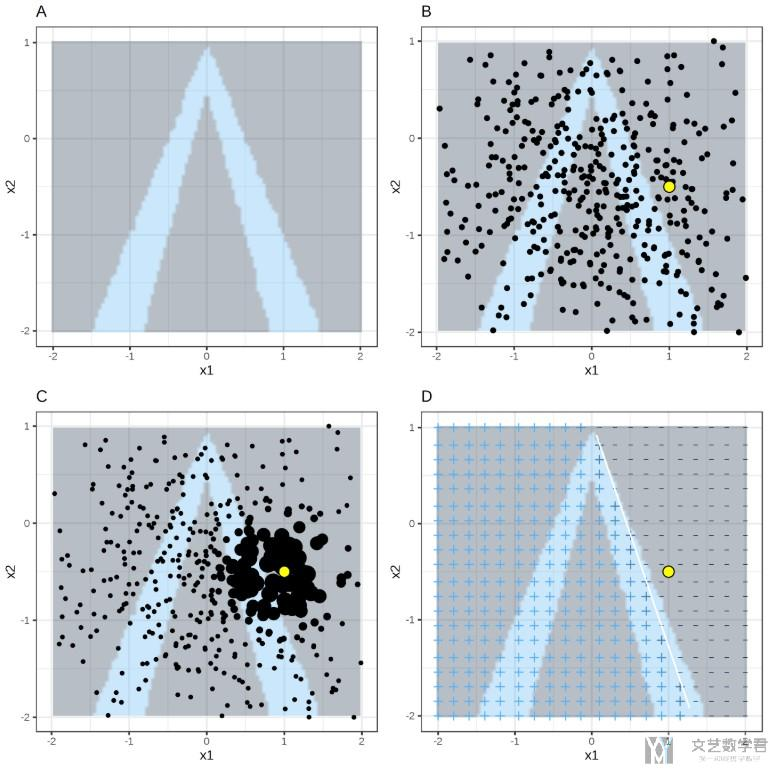• 图A表示: 随机森林的分类结果, 颜色深的为一类, 颜色浅的为一类.
• 图B表示: 通过对特征进行扰动得到的新的数据集.
• 图C表示: 对每一个数据进行加权.
• 图D表示: 对简单的模型进行求解.

## 对Pytorch搭建的模型进行解释

### 定义预测函数

1. # 定义分类器
2. def batch_predict(data, model=fullModel):
3.     """
4.     model: pytorch训练的模型
5.     data: 需要预测的数据
6.     """
7.     X_tensor = torch.from_numpy(data).float()
8.     model.eval()
9.     device = torch.device("cuda" if torch.cuda.is_available() else "cpu")
10.     model.to(device)
11.     X_tensor = X_tensor.to(device)
12.     logits = model(X_tensor)
13.     probs = F.softmax(logits, dim=1)
14.     return probs.detach().cpu().numpy()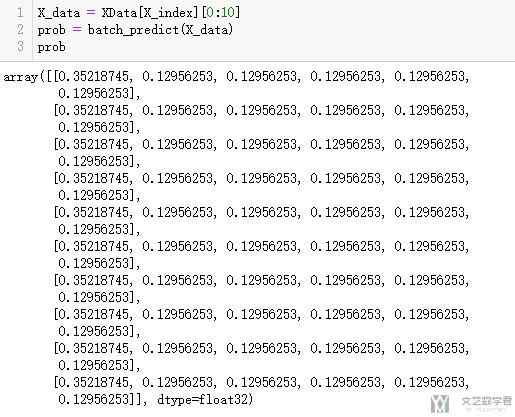### 对数据进行解释

1. # 创建解释器
2. import lime
3. import lime.lime_tabular

1. X_tensor = torch.from_numpy(XData[X_index]).float()
2. y_tensor = torch.from_numpy(np.array(y)).long()

1. targets =  ['back', 'land', 'neptune', 'pod', 'smurf', 'teardrop']
2. explainer = lime.lime_tabular.LimeTabularExplainer(XData[X_index],
3.                                                    feature_names=dfDataTrain.columns[:-4].values,
4.                                                   class_names=targets,
5.                                                   discretize_continuous=True)

1. # 解释某一个样本
2. exp = explainer.explain_instance(XData[X_index],
3.                                 batch_predict,
4.                                 num_features=5,
5.                                 top_labels=5)

1. exp.show_in_notebook(show_table=True, show_all=False)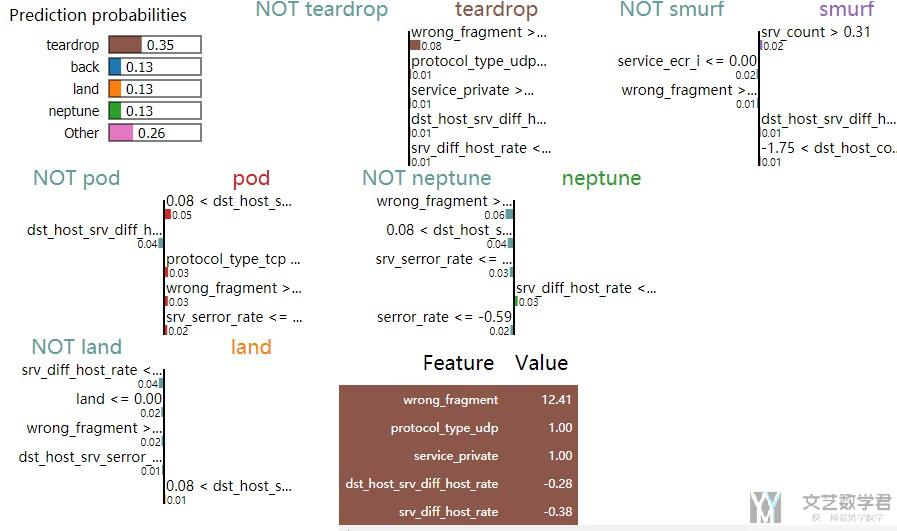1. exp.as_pyplot_figure(label=5)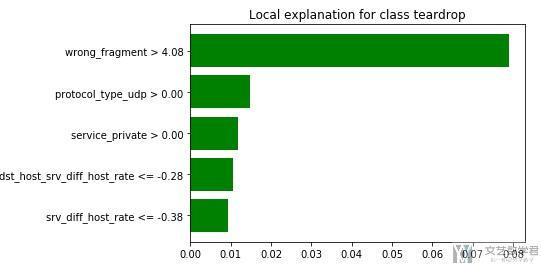• 微信公众号
• 关注微信公众号
•• QQ群
• 我们的QQ群号
•• 本文由 发表于 2019年9月23日07:01:20
• 转载请务必保留本文链接：https://mathpretty.com/11205.html